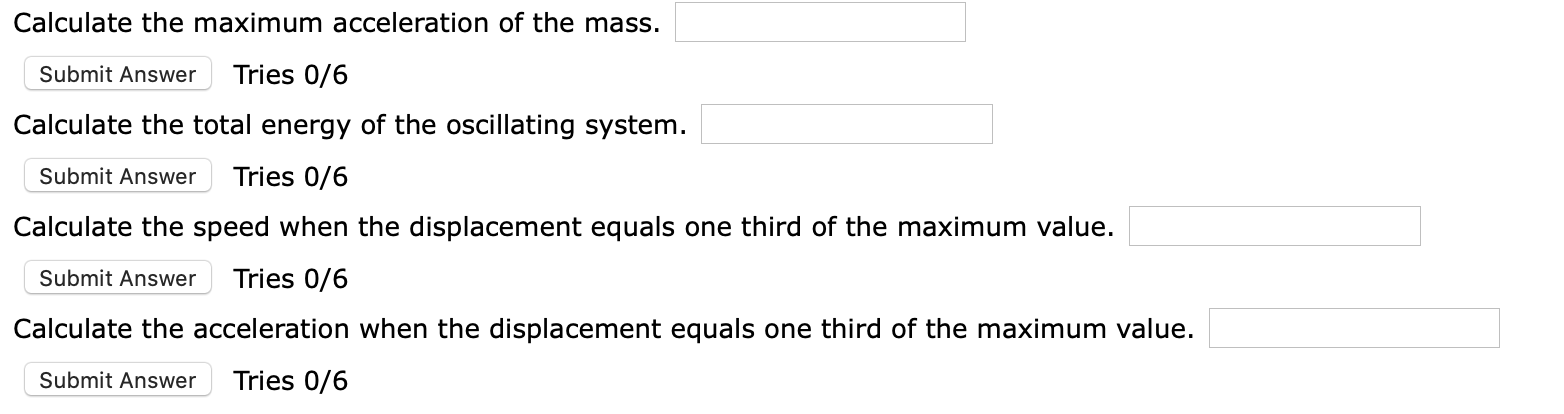# A 2.20 kg mass is attached to a spring and placed on a horizontal, smooth surface. A horizontal force of 22.7 N is required to hold the mass at rest when it is pulled 0.205 m from itsequilibrium position (the origin of the x axis). The mass is now released from rest with an initial displacement of xi0.205 m, and it subsequently undergoes simple harmonic oscillations. Calculate the maximum acceleration of the mass.Tries 0/6Submit AnswerCalculate the total energy of the oscillating system.Tries 0/6Submit AnswerCalculate the speed when the displacement equals one third of the maximum value.Tries 0/6Submit AnswerCalculate the acceleration when the displacement equals one third of the maximum value.Tries 0/6Submit Answer

Question
16 viewshelp_outlineImage TranscriptioncloseA 2.20 kg mass is attached to a spring and placed on a horizontal, smooth surface. A horizontal force of 22.7 N is required to hold the mass at rest when it is pulled 0.205 m from its equilibrium position (the origin of the x axis). The mass is now released from rest with an initial displacement of xi 0.205 m, and it subsequently undergoes simple harmonic oscillations. fullscreenhelp_outlineImage TranscriptioncloseCalculate the maximum acceleration of the mass. Tries 0/6 Submit Answer Calculate the total energy of the oscillating system. Tries 0/6 Submit Answer Calculate the speed when the displacement equals one third of the maximum value. Tries 0/6 Submit Answer Calculate the acceleration when the displacement equals one third of the maximum value. Tries 0/6 Submit Answer fullscreen
check_circle

Step 1

Since we are entitled to answer up to 3 sub-parts, we’ll answer the first 3 as you have not mentioned the subparts you need help with. Please resubmit the question and specify the other subparts you’d like to get answered.

Step 2

Given:

Mass = 2.20 kg

Force = 22.7 N

Displacement, x = 0.205 m

Step 3

Part (a):  maximum acce...

### Want to see the full answer?

See Solution

#### Want to see this answer and more?

Solutions are written by subject experts who are available 24/7. Questions are typically answered within 1 hour.*

See Solution
*Response times may vary by subject and question.
Tagged in

### Vibrations